# Mathnasium #MathTricks: Fractions (Comparing Fractions Part 1)

By Mathnasium | Added Apr 6, 2022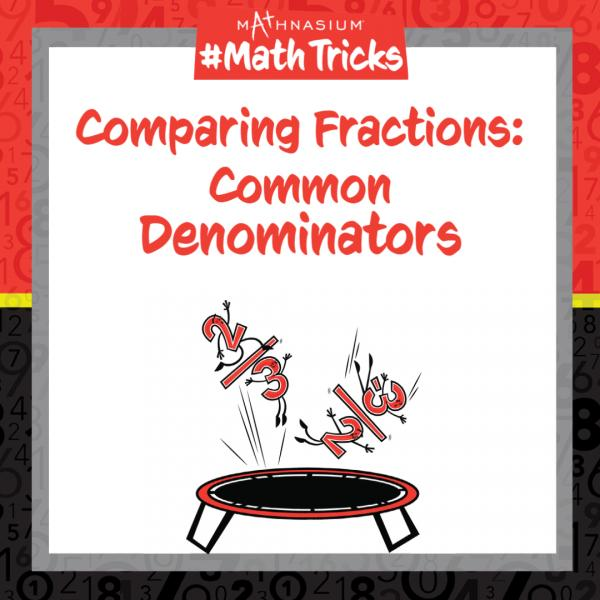Welcome to Mathnasium’s Math Tricks series. Kids commonly fear fractions when they encounter them. The goal is to group specific fractions and observe patterns. With these patterns, we can develop “tricks” to compare them. Today we are using our knowledge of the parts of a fraction to identify the biggest and smallest fractions with common denominators.

Fractions are composed of a numerator and a denominator. The first tells us how many parts of the whole we are considering – and is generally located above the “vincula” (bar) in the fraction. The latter (the denomination) tells us the total number of equal parts in the whole – and is generally located under the “vincula” (bar) in the fraction. Fractions with common denominators have “the same denomination, the same name,” so we only need to compare the numerators.

Follow the examples below to identify the biggest and smallest fractions.

# Example 1: You love pizza but you are sharing one with a group of friends. Would you rather have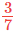,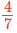, or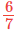of the pizza?

Step 1: Identify what you are looking for.

You love pizza and so you want the biggest fraction.

Step 2: Identify the name of each fraction given.

They are all sevenths.

Step 3: Compare the numerators.

6 is greater than 4 and 3.

Answer: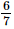67

# Example 2: Your friend does not like carrots. Do they prefer to have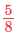or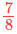of a carrot?

Step 1: Identify what you are looking for.

Your friend does not like carrots and so they want the smallest fraction.

Step 2: Identify the name of each fraction given.

They are all eighths.

Step 3: Compare the numerators.

5 is less than 7.

Answer: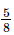58

Now, with this strategy, you are ready to compare fractions with common denominators. Click here for more practice problems.

If you missed this or any of our other Math Tricks videos, check them out on our YouTube channel!